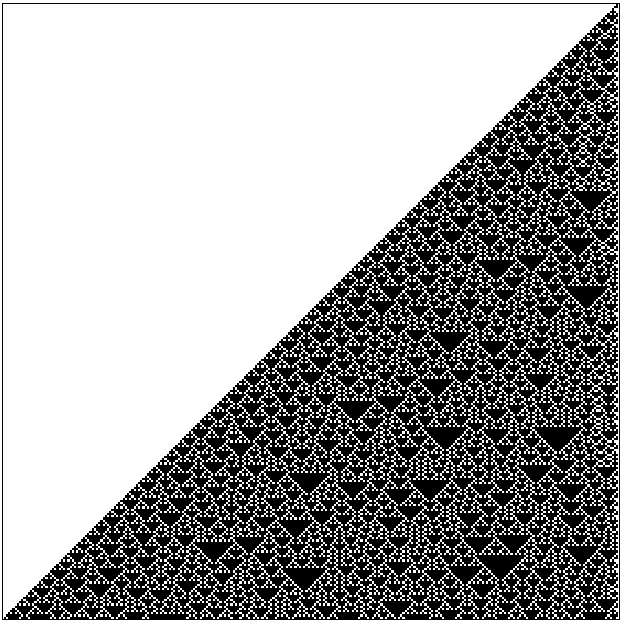Here’s some Matlab code for the particularly simple CA which Wolfram extensively classified and studied. The beauty of this code, and something I haven’t seen elsewhere, is that the function takes the Wolfram classification number as an argument (e.g. `wolfram(110);`), making it simple to investigate each of them.Example Output from the Code, showing Wolfram's Rule 182
``````function wolfram(wolfrule, initialstate, nrows)
% WOLFRAM    Displays evolution of a famous 1-D Cellular Automata.
%    Pick a rule from 1-256 (Wolfram's classification),
%    WOLFRAM(110) is the most famous, but many others are interesting:
%    rich=[18 30 45 73 89 101 102 105 109 110 126 129 135 ...
%        137 149 151 153 161 167 169 181 182 183 193 195 225];
%    Some rules die immediately with the default inital state, so
%    you must specify your own to see anything:
%    WOLFRAM(92, [1 0 0 0 0 0 0 0 0 0 1 0 1 0 0 0], 100);
%    Displaying all rules is interesting to appreciate the richness of
%    this CA's behaviour: for i=1:256, WOLFRAM(i), pause(0.5); end
%    Or jump to the rich ones: for i=rich, WOLFRAM(i), pause(2); end
%        Iain Haslam, April 2006.
if nargin < 3, nrows=700; end
if nargin < 2 %Use default initial state
ncols=700; A=zeros(nrows,ncols); A(1,ncols-1)=1;
else
[unused, ncols]=size(initialstate)
A=zeros(nrows, ncols); A(1,:)=initialstate;
end
rule=dec2bin(wolfrule,8);
for i=1:8
ru(i)=str2num(rule(i));
end
for i=2:nrows
for j=2:ncols-1
l=A(i-1,j-1); m=A(i-1,j); r=A(i-1,j+1);
if(( l & m & r & ru(1)) | ...
( l & m &~r & ru(2)) | ...
( l &~m & r & ru(3)) | ...
( l &~m &~r & ru(4)) | ...
(~l & m & r & ru(5)) | ...
(~l & m &~r & ru(6)) | ...
(~l &~m & r & ru(7)) | ...
(~l &~m &~r & ru(8)) )
A(i,j)=1;
end
end
end
colormap(gray(2)); image(2-A); axis image; title(wolfrule);``````